# 8 Examples of Simplifying Trig Expressions

Today, I’ll go over eight examples of simplifying trig expressions using division. We’ll look at how to reduce terms down to a single number or a single trigonometric function. These types of problems can be confusing because a rational expression is generally a fraction, and a lot of students struggle with fractions. As we solve each problem, we’ll look to the division property, which I’ll explain and reference in almost every example.

## Example #1: (cosθsecθ)/cotθ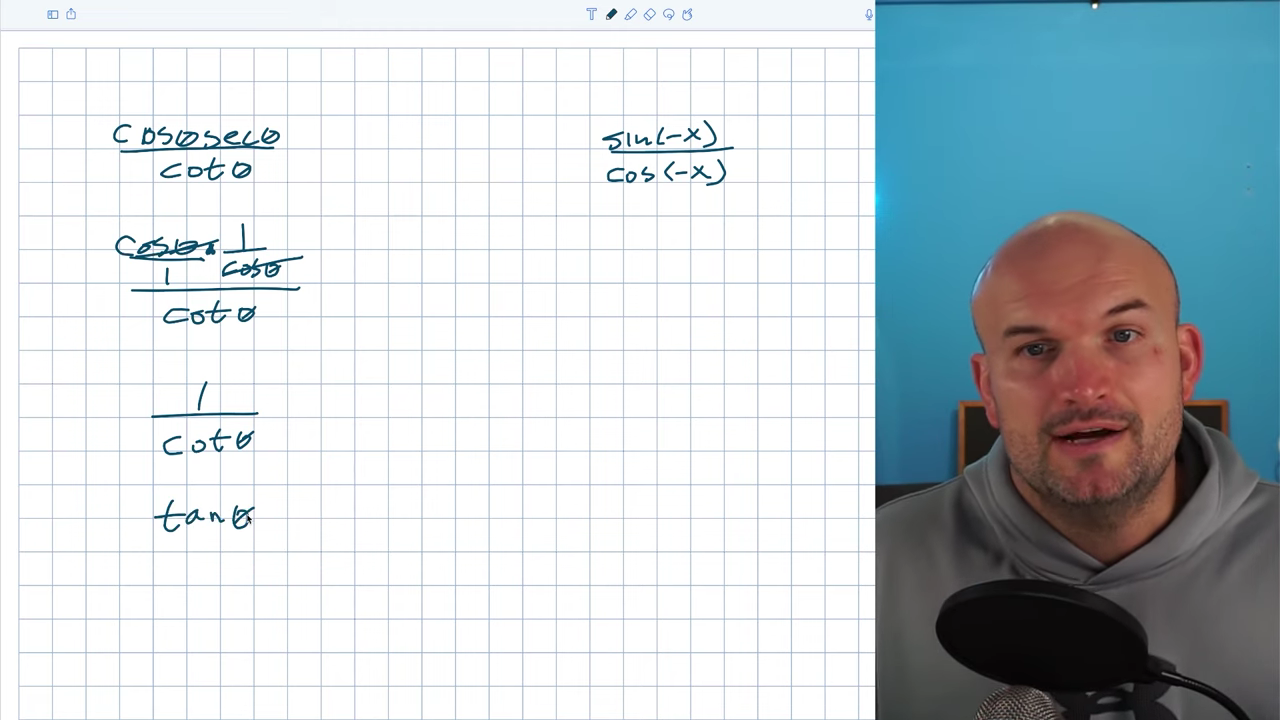In this example, I want to rewrite cosθsecθ in terms of sines and cosines so they’ll be reciprocals of one another, so their product will equal one. Now is a great time to review the division property, so I’ll include a picture of it written out. I’ll also reference it in other examples. According to the division property, whenever you have the same term in the numerator and the denominator separated by multiplication, they’ll divide to equal one. I’m left with 1 as the numerator, and my denominator is still cotθ. Using my reciprocal identity, I can simplify to tanθ.

## Example #2: sin(-x)/cos(-x)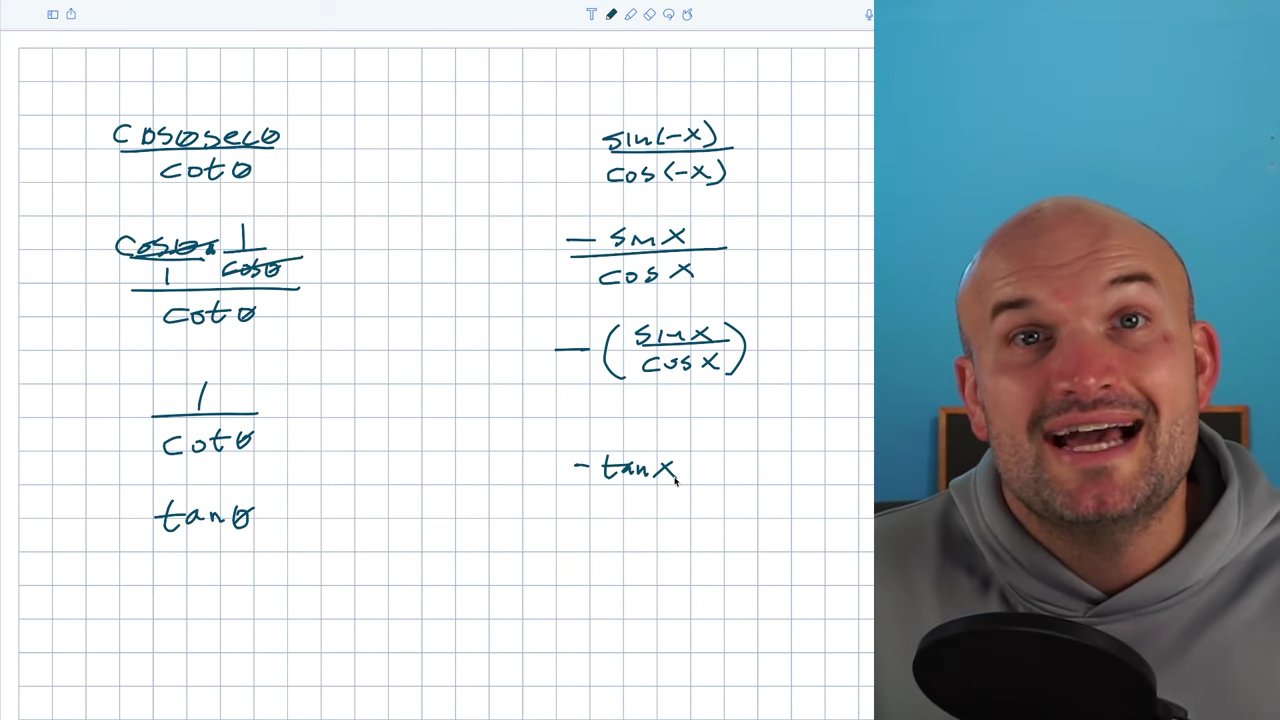The first thing I notice here is the even-odd identities. Think even-odd identities whenever you see a negative angle inside the argument of a trigonometric function. By applying even-odd identities, we can see that sin(-x) is equivalent to -sinx, and the cosine(-x) is equivalent to the positive cosine(x). Sometimes students see the negative in the numerator and get stuck, but we can put the negative in front as a product. It doesn’t matter if it’s in the numerator, the denominator, or just in front. I’ll use parentheses around (sinx/cosine), which, using the quotient identity, I know simplifies to tanx. My final answer is -tanx.

## Example #3: (sinθtanθ)/secθ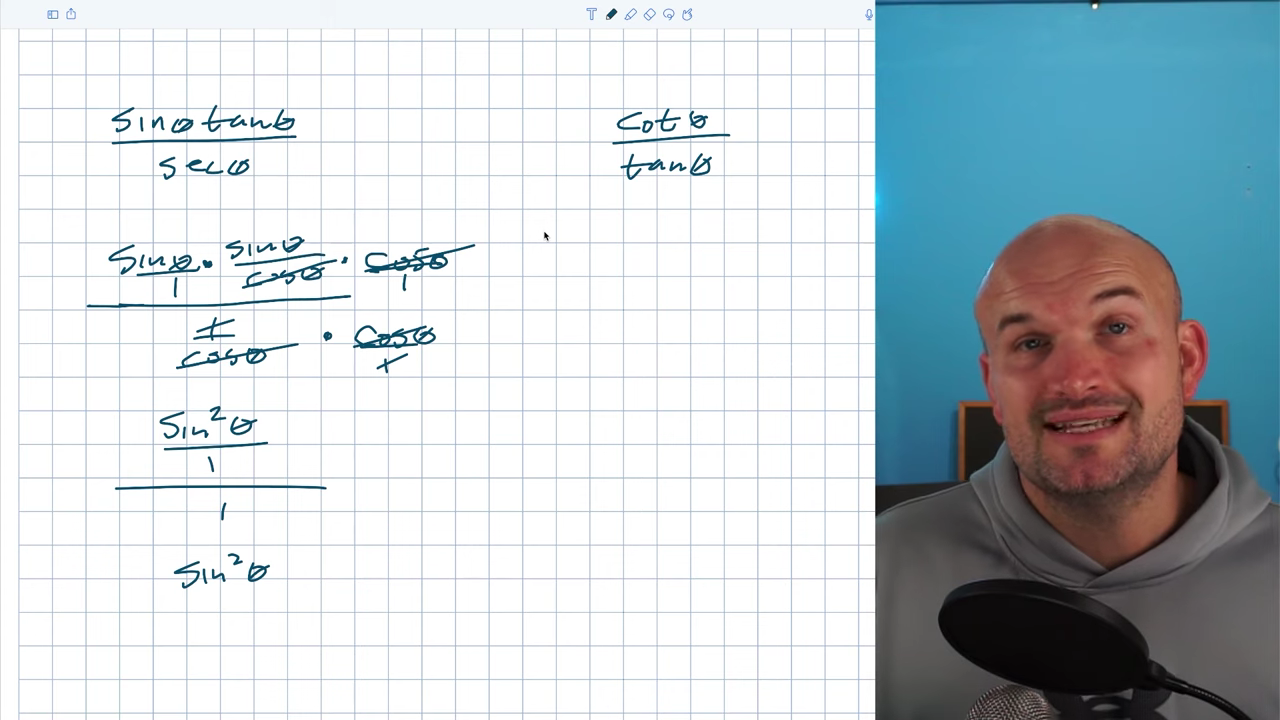In the next examples, we’ll add an extra step as we divide out terms. When I have a rational expression with the tangent or functions that aren’t in terms of sines and cosines, it’s easiest to rewrite the terms to see what can be divided out or what operations you can apply. In this example, the first thing I’ll do is rewrite tangent as sin/cos and secant as 1/cos. This step brought in more fractions, which we typically want to eliminate, but we also have an additional numerator and some denominators. Unfortunately, nothing simplifies out, but we can simplify the numerator to a fraction by multiplying sinθ by sinθ, giving us sin²θ. Sin²θ is divided by cosine, and the whole numerator is divided by 1/cosθ. To get rid of cosθ, I want to multiply by secθ.

We’ll use the division property to eliminate fractions. What can I multiply by that will divide with cosθ? In this case, cosθ is in the denominator, so I’ll multiply by cosθ on the top and the bottom to produce equivalent fractions. In the denominator, everything will multiply to equal 1. In the numerator, cosine is over 1, so I now have cosθ and cosθ that will divide to equal one. I’m left with (sin²θ/1)/1. Because everything is being divided by 1, we can write this as sin²θ.

## Example #4: cotθ/tanθ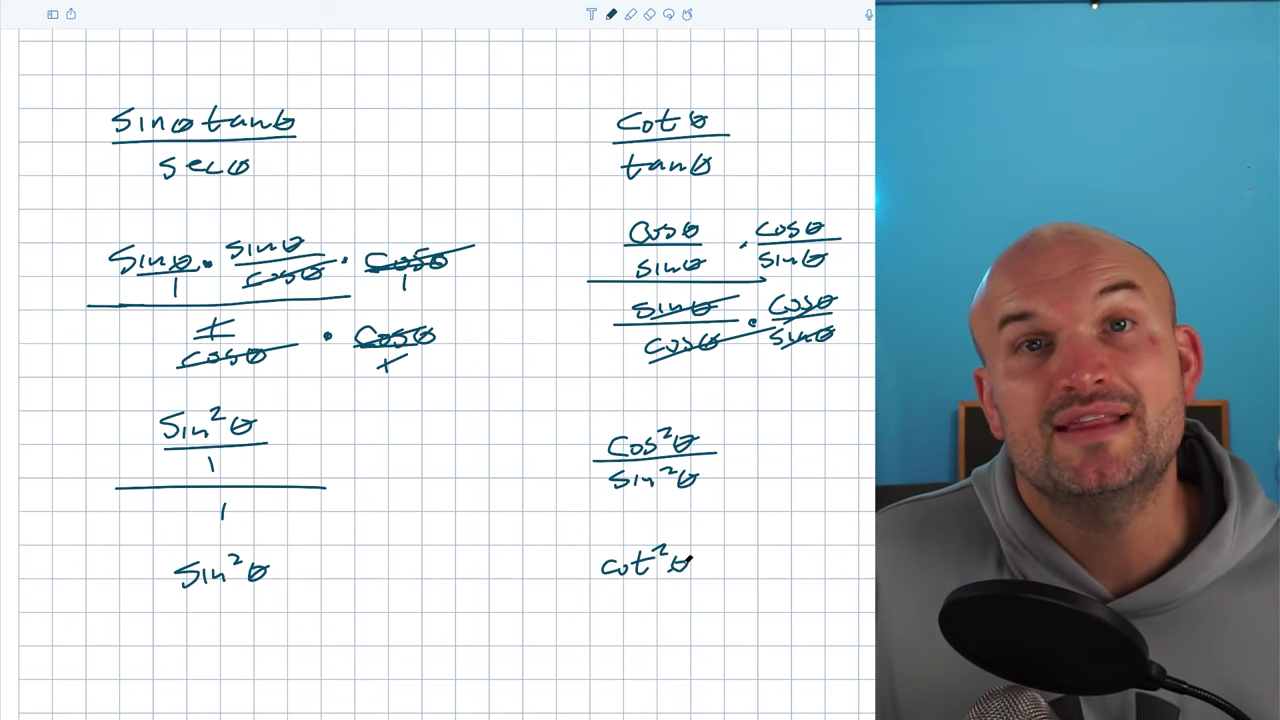I’ll start by rewriting this in terms of sines and cosines and then use the same process as the last example. Students will often get ‘cancel happy’ and start dividing things out when they see the same terms in the numerator and denominator, but you’ve got to be careful. In the last example, I multiplied by (cos/1) so that the cosines would divide out. We have a big denominator, so if I want to eliminate it, I need to make sure it will equal 1. The only way to do this is by multiplying by its reciprocal. The reciprocal of sinθ/cosθ is cosθ/sinθ. If I do this in the numerator, nothing divides out and I get cos ⋅ cos and sin ⋅ sin. My denominator will divide to equal 1 and my simplified answer is cos²θ/sin²θ, which can be simplified to cot²θ.

## Example #5: (sin²θ + cos²θ)/cos²θ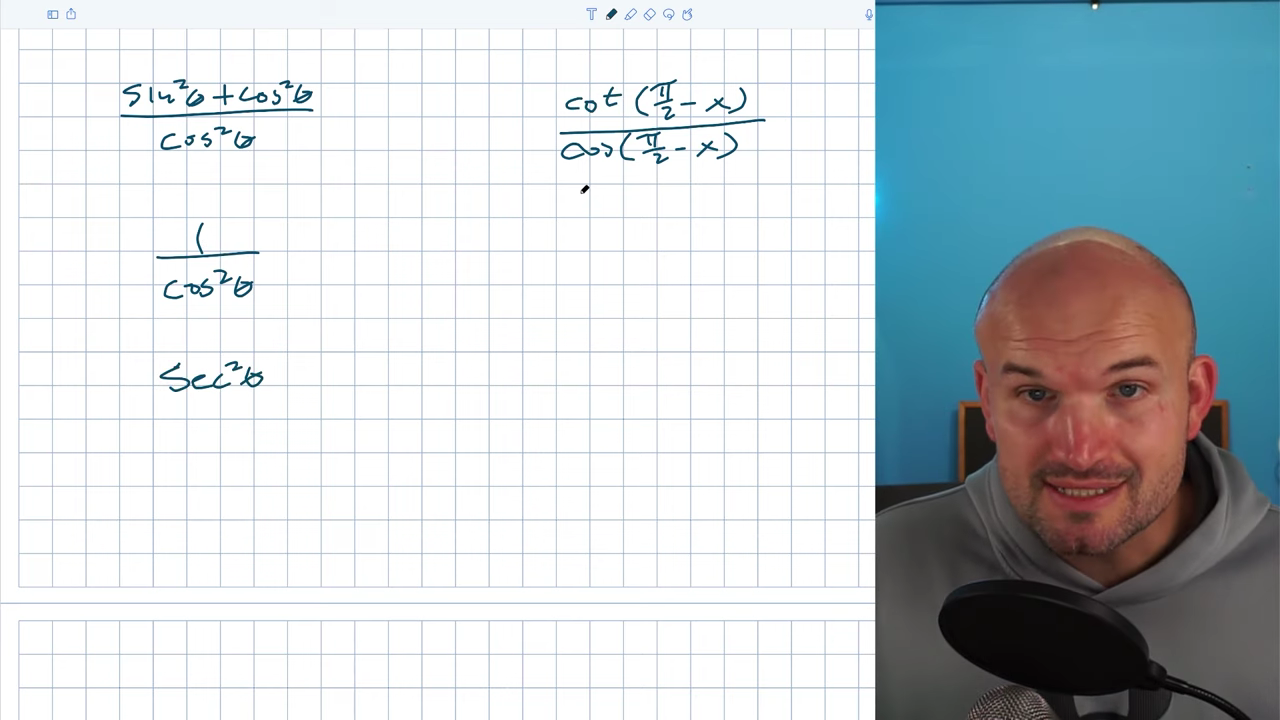When problems look more difficult than they need to be, we can make things simpler by using our identities. In the next two examples, we’ll talk about two different identities that make the problems look difficult but are actually fairly simple. In this example, a lot of students see the squares and get overwhelmed. Please don’t eliminate the cosines because the division property only works across multiplication, not addition and subtraction. Recognize the Pythagorean identity in the numerator. Sin²θ + cos²θ = 1, so I can simplify to 1/cos²θ. Using reciprocal identities, I recognize my answer as sec²θ.

## Example #6: (cot((π/2)-x))/(cos((π/2)-x))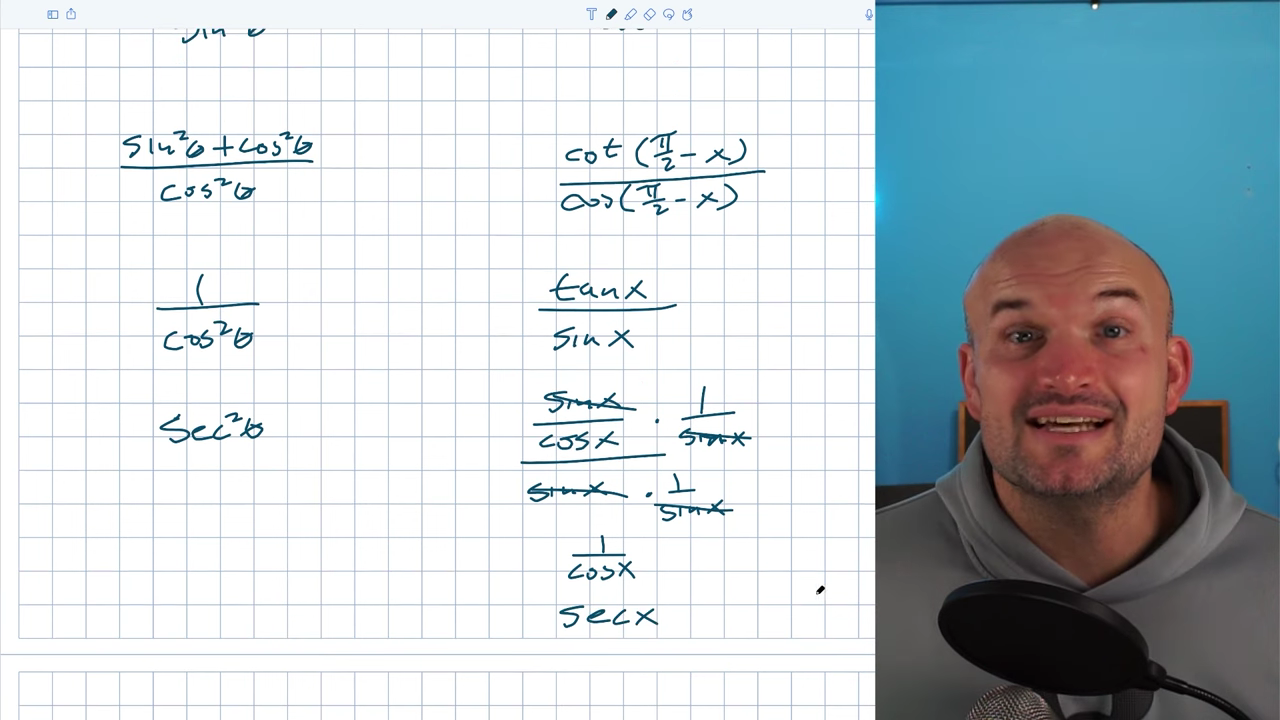There’s a lot going on in this example. What does π/2 – x represent? These are our co-function identities. I recommend either having a reference sheet accessible or refreshing your memory of them on a regular basis. They’re not very difficult to memorize and are useful to know. Cot(π/2 – x) is equal to tanx, and the cos(π/2 – x) is equal to the sinx. Now, I can write everything in terms of sines and cosines to better understand what’s going on.

I have (sinx/cosx)/sinx. I don’t want two different division bars, so I’ll get rid of the sinx in the denominator by multiplying by 1/sinx, which is also equal to cscx. When I multiply by sinx in the denominator and numerator, they divide out and give my denominator a 1. The sines divide out and leave me with (1/cos)/1, which is also equivalent to secx.

## Example #7: ((sec²θ – 1)/(secθ – 1)) -1In most of these examples, we can simplify the numerators by factoring. We often say to look for Pythagorean identities when working with trigonometric functions, but that’s not going to help much. In this example, we want to get rid of our denominator, so we’ll use the division property when looking for terms to divide out. We cannot simply divide out terms in the numerator and the denominator. I recognize this problem as the difference between two squares, so I can rewrite it as a secθ -1 ⋅ secθ + 1. Because these are separated by multiplication, I can apply the division property for the quantities of secθ – 1. I’m left with secθ +1 – 1, so my final answer is secθ.

## Example #8: (sinx – sinxcos²x)/cscx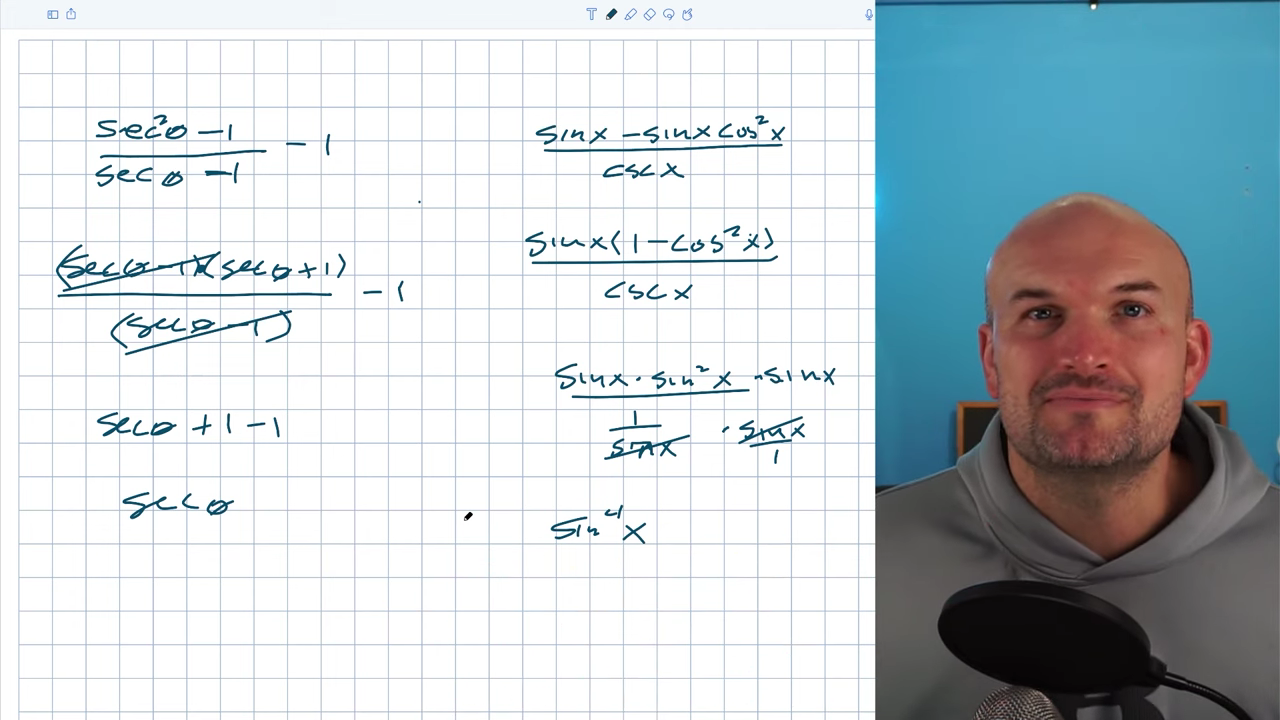This numerator is similar. I have a sinx in two expressions separated by subtraction, so I can factor it out. I could do the difference of two squares, but it won’t help me divide out my cscx or simplify the problem. Using my Pythagorean identities, I can rewrite this as sin²x. I also rewrote cosecant as 1/sinx. To get rid of 1/sinx or cscx, I’ll multiply by sinx in the numerator and denominator. This will bring my denominator to 1 because I have sinx in the numerator and the denominator. Multiplied together, my answer is sin^4x.

If this was helpful, feel free to comment and let me know! If you want more examples of how to simplify trigonometric expressions through division, click the link below. Cheers!